# Power Query – Formulas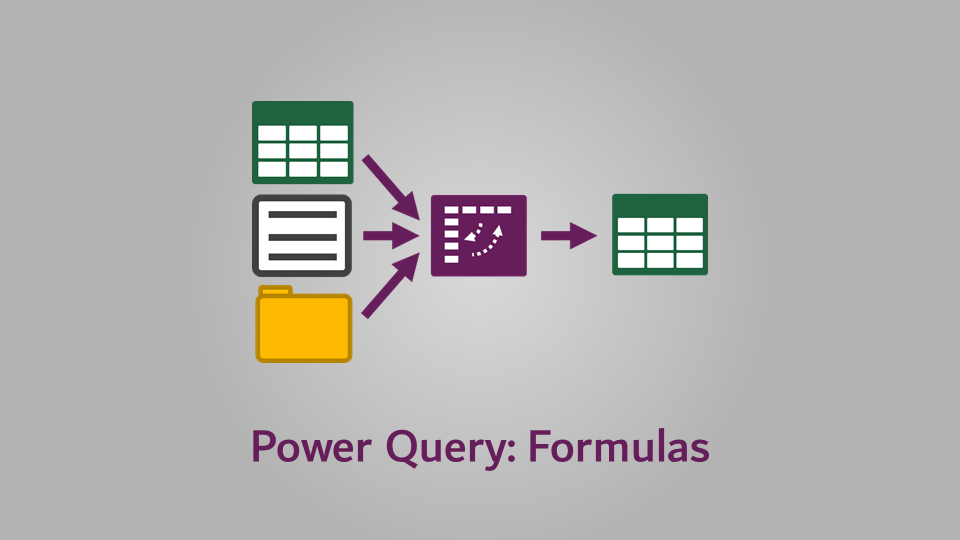Formulas are the lifeblood of Excel; they are essential to achieve even basic tasks. Power Query, on the other hand, has been designed so that most transformations are accessed through the intuitive user interface.  But Power Query has a formula language, with over 700 functions.  It is there so that we can tackle some of the most complicated situations we might encounter.

I am an Excel user at heart; therefore when I started using Power Query, I used lots of formulas, because that is what I did in Excel.  However, once I understood the interface, it became clear that most transformations can be achieved without any formulas at all.  Therefore, I recommend you look for transformations available from the standard menus before delving into specific functions.

One confusion is that functions are not the same as Excel’s; they are written using M code.  At the start, it’s frustrating that we transfer our existing knowled into Power Query. Once you understand how the formulas are constructed, you’ll soon realize that it’s easy to find what you need.  In this Power Query series, we have already used formulas every time we created a Custom Column.  This post will provide a better appreciation of how to use formulas.

## Writing a formula

Create a custom column by clicking  Add Column -> Custom Column.The Custom Column dialog box opens.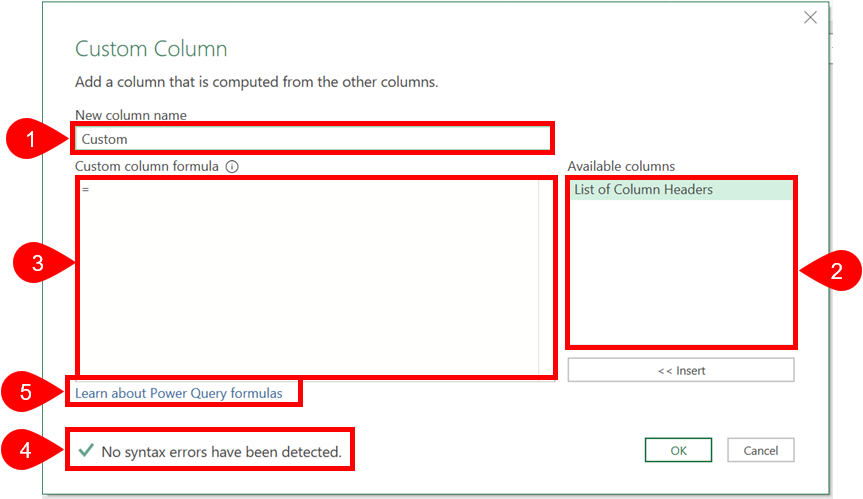The key areas of this dialog box are:

1. New column name
The text entered here is used as the column name in the data table.
2. Available columns
This contains a list of all the columns in the query.  Selecting a column and clicking on the Insert button (or double-clicking the name) will insert it into the formula.  This is exceptionally useful as it can reduce typos which will stop our code working as intended.
3. Custom column formula
This is where we enter the text which forms the formula.
4. Error check
The error check helps to let us know if the syntax is correct.  It will check if there are matching opening and closing brackets etc.  It will not tell you if the formula gives the right result, only that the syntax is correct.  Actually, it’s very difficult to interpret what the errors mean, therefore I work on the basis that green tick = good, and anything else = bad.
5. Learn about Power Query formulas
This link takes us to Microsoft’s Power Query formulas web pages.  If you get stuck, this is the place to go.

### Simple formula operations

Simple formula operations are similar to Excel:

`=[Column 1] + [Column 2]`

Subtraction

`=[Column 1] - [Column 2]`

Multiplication

`=[Column 1] * [Column 2]`

Division

`=[Column 1] / [Column 2]`

Concatenation

`=[Column 1] & [Column 2]`

Brackets / Parentheses

Parentheses or Brackets (depending on what you call them) work the same as in Excel.

`=([Column 1] + [Column 2]) / [Column 3]`

Equals

`=[Column 1] = [Column 2]`

The formula above will give a True or False result.

You do not need to enter the equals symbol ( = ) at the start; it will be in the formula box automatically.

Power

The Power operator ( ^ ) which we use in Excel does not work in Power Query formulas. Instead, you have to use the Number.Power formula.

Formula example #1

The screenshot below shows some of these basic formula operations in action.You can see the column names being used; Number #1 is divided by the total of Number #1  + Number #2.

Formula example #2

The screenshot below shows that formulas can be constructed from static values.  A formula can also include a mix of columns and static values.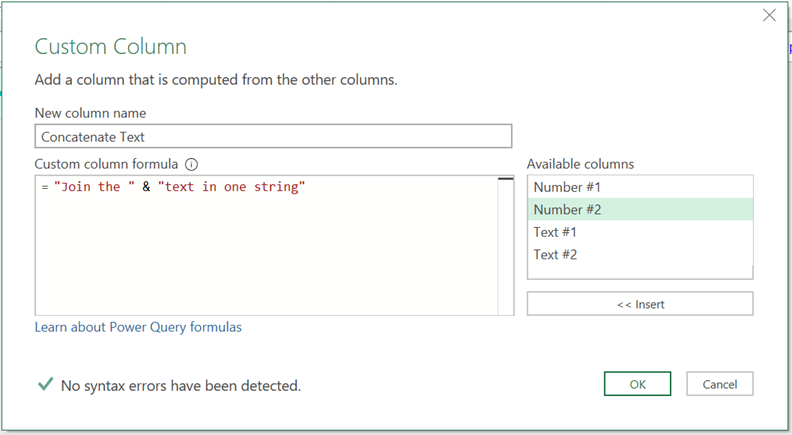### Row Context

Unless you do some advanced formula magic, the formulas in Power Query have what is known as “Row Context”.  This means the formula is applied to each row one by one.  You may show column names in the formula, however you are not applying this formula to complete columns, but to each row.

### Data types

Power Query formulas are picky about data types.  Functions expect certain data types to operate correctly.  For example, the Date.Year function returns the year from a date. If the column used within the function is not a date type, the formula will calculate to an error.  So, if the data type does not match the formula requirements we need to find a way to change it.  We can either add a step before creating the custom column, or we can use a converting formula.

Some of the most common converting formulas are:

• Text.From – To convert anything to text
• Date.ToText – To convert a date to text
• Date.From – To convert a number into a date
• Date.FromText – To convert text into a date
• Number.ToText – To convert a number to text
• Number.From – To convert any inputs to a number
• Number.FromText – To convert text into a number
• Logical.From – To convert numbers into their True or False values
• Logical.FromText – To convert text strings of “True” or “False” into boolean values of True or False
• Logical.ToText – To convert Boolean True or False values into “True” or “False” text strings

Formula Example #3

The following table contains numbers and text.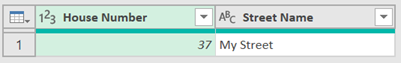As the House Number is a number, we need to convert it into text before we can combine it with Street Name into a single Address string.

The formula text would be:

`=Text.From([House Number]) & " " & [Street Name]`

The bold text highlights the use of the Text.From function to convert the number into a Text data type, before it is combined with the Street Name column.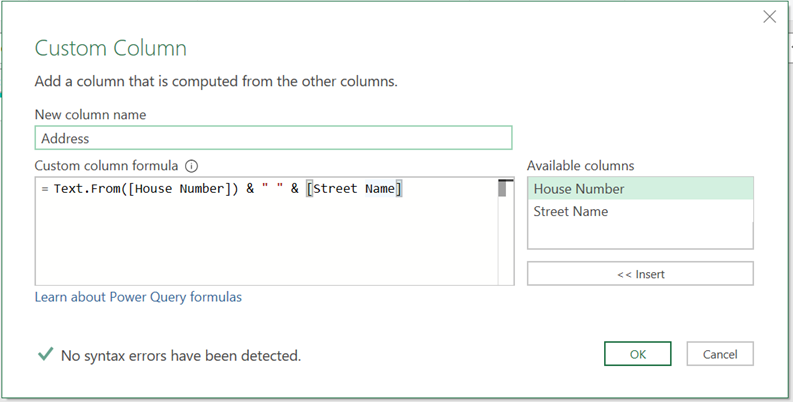## Finding Functions

As I said earlier, we cannot use Excel formulas.  The easiest ways to find the available formulas is to click on the Learn about Power Query formulas link.

The functions are grouped by what they do.  If we want a formula which outputs a Number, we would look at the Number group.  Most of the formulas in this section start with the word Number.  Here are some examples:

• Number.Abs – Returns the absolute value of a number
• Number.FromText – Returns a number from a text string
• Number.IsOdd – Returns true if the value is odd, or false if it is even.

• Decimal.From – Returns a decimal from a given value
• Percentage.From – Returns a percentage from a given value

Generally, formulas which output a date start with Date in the formula name (such as Date.Year), and formulas which output text start with Text in the formula name (such as Text.Start).

Think about Excel functions, how did you learn them?  You probably had someone show you, or you had to do some guessing with a lot of trial and error.  Because of how Power Query functions are named, it is much easier to find the function we want.

### IntelliSense

At the time of writing, Microsoft has recently released IntelliSense into Power Query.  This means we can start typing the name of a function and a list of options will appear.  The screenshot below shows the list of functions just by typing “Tex” into the formula box.IntelliSense helps us to discover functions, but also makes them significantly more usable.  After you’ve got the name of the function, type the opening bracket and it will show the arguments and data types required.  It’s taken a while for Power Query to get the functionality, but now that it’s here, I couldn’t live without it.### Looking at existing transformation steps

The transformations we use through the standard interface make use of the same functions as we have access to.

Let’s say we wanted to extract the first 4 letters from a text string.  We could use Add Column -> Extract -> First Characters from the Ribbon, then insert 4 into the Insert First Character dialog box.  We could then take a look at the M code in the Formula Bar for that applied step, it would show the following

`= Table.AddColumn(#"Changed Type", "First Characters", each Text.Start([Text Column], 4), type text)`

The bold section is the function Text.Start.  This function extracts the first characters from a text string (it is similar to Excel’s LEFT function).

This illustrates that any time you’re stuck, looking at the M code for similar transformations may give you a guide as to the type of function you might need.

## Essential formula tips

The differences between M code and Excel Functions can trip us up.  Some of the key differences are:

### M code is case sensitive

In Excel, we can write functions in upper or lower case, Excel understands what we want and will convert functions to upper case for us.  Power Query in the past has not been so forgiving; Text.Start is the name of a function, while text.start is meaningless as it’s using the wrong case for each word.  In more recent versions, Power Query has started to convert functions into the correct case.  As this is a new feature, you may not have it yet.

### M starts counting at 0

Text.PositionOf is a function which finds the position of a string within another string.  If we use the following M code as an example:

`=Text.PositionOf("Excel Off The Grid","Excel")`

This is similar to Excel’s FIND function with the arguments in the opposite way round.

`=FIND("Excel","Excel Off The Grid")`

The Excel function returns a value of 1, while the Power Query function returns a value of 0.  This is because Power Query starts counting from 0.  It may seem odd to most Excel users, but it is common in the world of programming languages.

## Practice, practice, practice

In this post, we’ve covered the basics of Power Query formulas.  I guarantee that unless you practice this over the next few weeks or months that you will forget everything you’ve read.  Practice is essential.  M is similar to Excel, therefore you’ll need to regularly refer to the M function library to find what you need.  There is no shame in that… actually, that’s the whole point of it being there.

That’s enough talk from me, it’s time you went and tried some of these out for yourself.Get our FREE VBA eBook of the 30 most useful Excel VBA macros.
Automate Excel so that you can save time and stop doing the jobs a trained monkey could do.

Email Address * First Name *

Don’t forget:

I’m guessing the examples in this post didn’t exactly meet your situation.  We all use Excel differently, so it’s impossible to write a post that will meet everybody’s needs.  By taking the time to understand the techniques and principles in this post (and elsewhere on this site) you should be able to adapt it to your needs.

But, if you’re still struggling you should:

1. Read other blogs, or watch YouTube videos on the same topic.  You will benefit much more by discovering your own solutions.
2. Ask the ‘Excel Ninja’ in your office.  It’s amazing what things other people know.
3. Ask a question in a forum like Mr Excel, or the Microsoft Answers Community.  Remember, the people on these forums are generally giving their time for free.  So take care to craft your question, make sure it’s clear and concise.  List all the things you’ve tried, and provide screenshots, code segments and example workbooks.
4. Use Excel Rescue, who are my consultancy partner.   They help by providing solutions to smaller Excel problems.

What next?
Don’t go yet, there is plenty more to learn on Excel Off The Grid.  Check out the latest posts:

## 2 thoughts on “Power Query – Formulas”

1.sonny says:

I want to add a column to the new created sheet. I have a macro where the rows from the main get delated if the date expires, the problem I’m having is when I refresh in the new sheet, that data get’s deleted but not the row from the columns I added (that had custom data)

•Excel Off The Grid says:

Sonny – I’m not sure I understand the question, or how it relates to the topic of Power Query Formulas. Can you provide more informaiton?